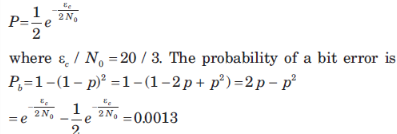Courses

# Test: Biasing In BJT Amplifier Circuits & Spread Spectrum

## 18 Questions MCQ Test Electronic Devices | Test: Biasing In BJT Amplifier Circuits & Spread Spectrum

Description
This mock test of Test: Biasing In BJT Amplifier Circuits & Spread Spectrum for Electrical Engineering (EE) helps you for every Electrical Engineering (EE) entrance exam. This contains 18 Multiple Choice Questions for Electrical Engineering (EE) Test: Biasing In BJT Amplifier Circuits & Spread Spectrum (mcq) to study with solutions a complete question bank. The solved questions answers in this Test: Biasing In BJT Amplifier Circuits & Spread Spectrum quiz give you a good mix of easy questions and tough questions. Electrical Engineering (EE) students definitely take this Test: Biasing In BJT Amplifier Circuits & Spread Spectrum exercise for a better result in the exam. You can find other Test: Biasing In BJT Amplifier Circuits & Spread Spectrum extra questions, long questions & short questions for Electrical Engineering (EE) on EduRev as well by searching above.
QUESTION: 1

### (Q.1-Q.6) consider the figure shown below and answer the questions that proceed.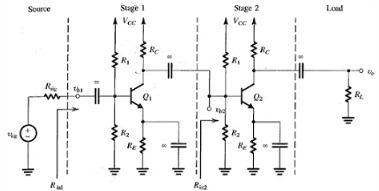For Vcc = 15V, R1 = 100 kΩ, R(E) = 3.9 kΩ, R(C) = 6.8 kΩ and ß = 100, determine the dc collector current for each transistor.

Solution: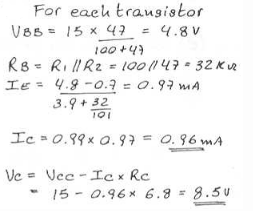QUESTION: 2

### For Vcc = 15V, R1 = 100 kΩ, R(E) = 3.9 kΩ, R(C) = 6.8 kΩ and ß = 100, determine the dc collector voltage for each transistor.

Solution: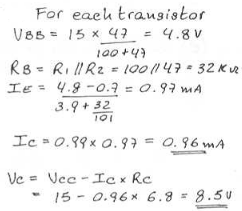QUESTION: 3

### Find R (in 1) for R (sig) = 5 kΩ.

Solution:

It is the parallel combination of the 32 kΩ resistor and 2.6 kΩ resistor respectively.

QUESTION: 4

Find Vb1/Vsig for R(sig) = 5 kΩ.

Solution: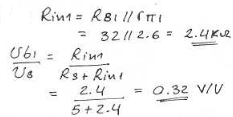QUESTION: 5

Find R (in 2).

Solution:

It is the parallel combination of the 32 kΩ resistor and 2.6 kΩ resistor respectively.

QUESTION: 6

Find Vb2/Vb1.

Solution: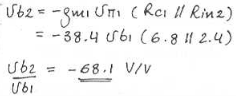QUESTION: 7

For Rl = 2 kΩ find Vo/Vb2.

Solution: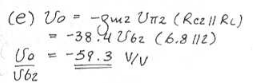QUESTION: 8

Find the overall voltage gain.

Solution: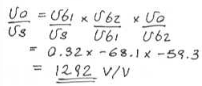QUESTION: 9

(Q.1-Q.3) A pseudo-noise (PN) sequence is generated using a feedback shift register of length m = 4. The chip rate is 107 chips per second.

Q. The PN sequence length is

Solution:

The PN sequence length is N = 2m – 1 = 16 – 1 = 15.

QUESTION: 10

The chip duration is

Solution:

Tc = 1/(107) or 0.1 µs.

QUESTION: 11

The period of PN sequence is

Solution:

The period of the PN sequence is T = NTc = 15 x 0.1 = 1.5 s

QUESTION: 12

A slow FH/MFSK system has the following parameters.
Number of bits per MFSK symbol = 4
Number of MFSK symbol per hop = 5
The processing gain of the system is

Solution:

PG = Wc/Rs = 5 x 4 = 20 or 26 db.

QUESTION: 13

A fast FH/MFSK system has the following parameters.
Number of bits per MFSK symbol = 4
Number of pops per MFSK symbol = 4
The processing gain of the system is

Solution:

PG = 4 x 4 = 16 or 12 db.

QUESTION: 14

(Q.6-Q.7) A rate 1/2 convolution code with dfrec = 10 is used to encode a data sequence occurring at a rate of 1 kbps. The modulation is binary PSK. The DS spread spectrum sequence has a chip rate of 10 MHz.

Q. The coding gain is

Solution:

0.5 x 10 = 5 or 7 db is the coding gain.

QUESTION: 15

The processing gain is

Solution:

PG = (107)/(2 x 1000) = 5000 or 37 db.

QUESTION: 16

(Q.8-Q.9) An FH binary orthogonal FSK system employs an m 15 stage liner feedback shift register that generates an ML sequence. Each state of the shift register selects one of L non over lapping frequency bands in the hopping pattern. The bit rate is 100 bits/s. The demodulator employ non coherent detection.[/expand]

Q.  If the hop rate is one per bit, the hopping bandwidth for this channel is

Solution:

The length of the shift-register sequence is L = 2m – 1215 = 32767 bits
For binary FSK modulation, the minimum frequency separation is 2/T, where 1/T is the symbol (bit) rate. The hop rate is 100 hops/sec. Since the shift register has L 32767 states and each state utilizes a bandwidth of 2/T = 200 Hz, then the total bandwidth for the FH signal is 6.5534 MHz.

QUESTION: 17

Suppose the hop rate is increased to 2 hops/bit and the receiver uses square law combining the signal over two hops. The hopping bandwidth for this channel is

Solution:

If the hopping rate is 2 hops/bit and the bit rate is 100 bits/sec, then, the hop rate is 200 hops/sec. The minimum frequency separation for orthogonality 2/T 400 Hz. Since there are N 32767 states of the shift register and for each state we select one of two frequencies separated by 400 Hz, the hopping bandwidth is 13.1068 MHz.

QUESTION: 18

In a fast FH spread spectrum system, the information is transmitted via FSK with non coherent detection. Suppose there are N = 3 hops/bit with hard decision decoding of the signal in each hop. The channel is AWGN with power spectral density 0.5No and an SNR 20 ~13 dB (total SNR over the three hops). The probability of error for this system is

Solution:

The total SNR for three hops is 20 ~ 13 dB. Therefore the SNR per hop is 20/3. The probability of a chip error with non-coherent detection is